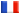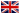# Séminaire de Cryptographie

## Juergen Klueners### Constructive Galois Theory

In constructive Galois theory, there are two main questions: the direct problem and the inverse problem. For the inverse problem the question is whether it is possible to find a polynomial such that the Galois group of that polynomial is a given finite group. In this talk, we will focus on the direct problem. Given a polynomial f we explain how to compute the Galois group of this polynomial. The presented algorithms are implemented in KASH and work up to degree 23 for polynomials over number fields and function fields (in one variable).

In a second part of the talk, we report on a database containing number fields up to degree 15. The database is complete in the sense that for each transitive group up to degree 15 there is at least one polynomial in the database which has this given Galois group.